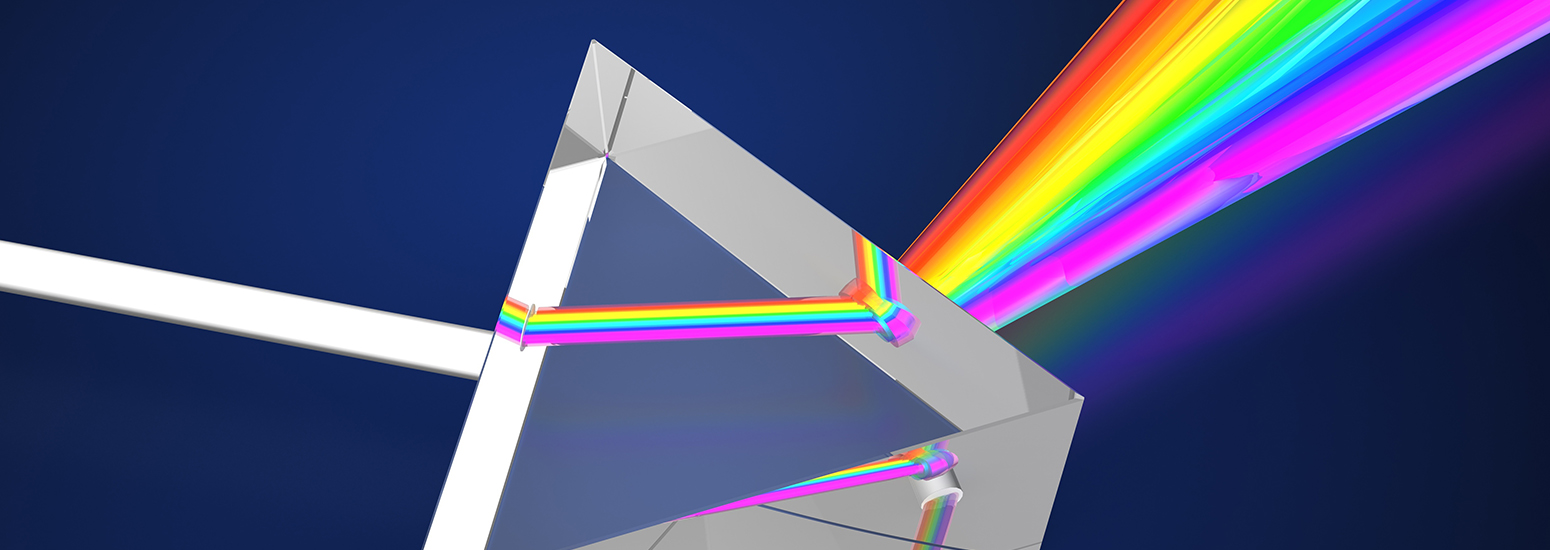# Theory of Raman Scattering

When considering Raman scattering, we can think about the physics in one of two ways: the classical wave interpretation or the quantum particle interpretation. In the classical wave interpretation, light is considered as electromagnetic radiation, which contains an oscillating electric field that interacts with a molecule through its polarizability. Polarizability is determined by the electron cloud’s ability to interact with an electric field. For example, soft molecules such as benzene tend to be strong Raman scatterers while harder molecules like water tend to be fairly weak Raman scatterers.Figure R-2 Comparison of Raman Scattering Interpretations

When considering the quantum particle interpretation, light is thought of as a photon which strikes the molecule and then inelasticaly scatters. In this interpretation the number of scattered photons is proportional to the size of the bond. For example, molecules with large Pi bonds such as benzene tend to scatter lots of photons, while water with small single bonds tends to be a very weak Raman scatterer. Figure R-2 shows a visual comparison of the two methods.

When deriving the Raman effect, it is generally easiest to start with the classical interpretation by considering a simple diatomic molecule as a mass on a spring (as shown in figure R-3) where m represents the atomic mass, x represents the displacement, and K represents the bond strength.Figure R-3 Diatomic Molecule as a Mass on a Spring

When using this approximation, the displacement of the molecule can be expressed by using Hooke’s law as,Equation R-1

By replacing the reduced mass (m1m2/[m1+m2]) with μ and the total displacement (x1+x2) with q, the equation can be simplified to,Equation R-2

By solving this equation for q we get,Equation R-3

where νm is the molecular vibration and is defined as,Equation R-4

From equations R-3 and R-4, it is apparent that the molecule vibrates in a cosine pattern with a frequency proportional to the bond strength and inversely proportional to the reduced mass. From this we can see that each molecule will have its own unique vibrational signatures which are determined not only by the atoms in the molecule, but also the characteristics of the individual bonds. Through the Raman effect, these vibrational frequencies can be measured due to the fact that the polorizability of a molecule, α, is a function of displacement, q. When incident light interacts with a molecule, it induces a dipole moment, P, equal to that of the product of the polorizability of the molecule and the electric field of the incident light source. This can be expressed as,Equation R-5

where Eo is the intensity and νo is the frequency of the electric field. Using the small amplitude approximation, the polorizability can be described as a linear function of displacement,Equation R-6

which when combined with equations R-3 and R-5 results in,Equation R-7

In Equation R-7 we see that there are two resultant effects from the interaction of the molecule and the incident light. The first effect is called Rayleigh scattering, which is the dominate effect and results in no change in the frequency of the incident light. The second effect is the Raman scattered component and when expanded to,Equation R-8

can be shown to shift the frequency of the incident light by plus or minus the frequency of the molecular vibration. The increase in frequency is known as an Anti-Stokes shift and the decrease in frequency is known as a Stokes shift. By measuring the change in frequency from the incident light (typically only the Stokes shift is used for this measurement) the Raman effect now gives spectroscopists a means of directly measuring the vibrational frequency of a molecular bond.

Now that we have derived the Raman effect using the classical wave interpretation, we can use the quantum particle interpretation to better visualize the process and determine additional information. As discussed earlier in the quantum interpretation, the Raman effect is described as inelastic scattering of a photon off of a molecular bond. From the Jablonski diagram shown in figure R-4, we can see that this results from the incident photon exciting the molecule into a virtual energy state.Figure R-4 Jablonski Diagram Representing
Quantum Energy Transitions for Rayleigh and Raman Scattering

When this occurs, there are three different potential outcomes. First, the molecule can relax back down to the ground state and emit a photon of equal energy to that of the incident photon; this is an elastic process and is again referred to as Rayleigh scattering. Second, the molecule can relax to a real phonon state and emit a photon with less energy than the incident photon; this is called Stokes shifted Raman scattering. The third potential outcome is that the molecule is already in an excited phonon state, is excited to a higher virtual state, and then relaxes back down to the ground state emitting a photon with more energy than the incident photon; this is called Anti-Stokes Raman scattering. Due to the fact that most molecules will be found in the ground state at room temperature, there is a much lower probability that a photon will be Anti-Stokes scattered. As a result, most Raman measurements are performed considering only the Stokes shifted light.

By further investigating the quantum interpretation of the Raman effect, it can be shown that the power of the scattered light, Ps, is equal to the product of the intensity of the incident photons, Io, and a value known as the Raman cross-section, σR. It can be shown that,Equation R-9

where λ equals the wavelength of the incident photon. Therefore,Equation R-10

From equation R-10 it is clear that there is a linear relationship between the power of the scattered light and the intensity of the incident light as well as a relationship between the power of the scattered light and the inverse of the wavelength to the fourth power. Therefore, it would appear that it is always desirable to use a short excitation wavelength and a high power excitation source based on these relationships. However, as we will see in the next section, this is not always the case.